Eth: 0x00cce8E2e56a543abc084920eee3f88eFD0921ea

## Sunday, March 4, 2012

### If Statement in Matlab

How to do a double if statement in Matlab (have a process execute under 2 or more conditions):

Matlab 2011a (and other versions) is tricky program to code correctly. Sometimes you think you are doing everything right and you are not getting any warning messages but things are still broken. It is easy to do this with the IF statement. Recently I coded up a double or compound if statement and I thought it was working properly. I had the script:

However, Matlab 2011a will not check the second condition! Furthermore, it won't tell you there is a problem. The correct way to code this up to make sure both conditions are checked is to either brute force the problem by building in two if statements:

Or the more refined version, use the && operator to make sure both conditions are evaluated:

Read more on IF statements at Matlab documentation site.

Happy Coding
-Láscal

## Thursday, March 1, 2012

### How to Calculate Drag in LEO using Matlab

I'm going to highlight a simple model that can be used to calculate drag in Low Earth Orbit (LEO). Objects in orbit around earth experience atmospheric drag at altitudes of up to 1200km off the surface of the Earth. Spacecraft that orbit in LEO must compensate for drag or else their orbit will decay and they will burn up in the atmosphere. Anything that travels below 100km altitude is now so low that it will eventually either burn up or hit the surface of the planet. Drag is a complex force and there are many variables that one should take into account. However, we have simplified it down to the bare bones so you can understand the essentials in a simplified model.

Things you will need:
Data on your spacecraft you want to calculate drag on including: orbit altitude (assume circular orbit), surface area (assume spherical), mass of spacecraft, and the velocity of the spacecraft. Optional: A copy of Matlab to run example code.

Example Problem:
For our example we will assume an altitude of 455 (km). From the velocity equation of a circular orbit Vcirular=sqrt(mu/radius). Where mu for the Earth is 398,600 (km^3/s^2). Radius = (altitude + radius of earth) = 455 (km) + 6378 (km) = 6833 (km).  Our velocity is therefore 7.64 (km/s) = 7640 (m/s). We will assume a spacecraft mass of 150 (kg). Furthermore we will assume our spacecraft is a sphere with a diameter of 5 (meters).

Drag Equation:
Where p is the density of the atmosphere at that altitude, Cd is the coefficient of drag, V is our velocity and Area is the frontal area exposed to drag which is 1/2 the area of a sphere for our example. A good place to start is assuming a coefficient of drag of 2 to 2.2. This is typical of hypervelocity trajectories at the altitudes we are dealing with. Our velocity is 7640 (m/s) and our area for the front half of a sphere with a diameter of 5m is 39.26 (m^2). That leaves the density which is the most complicated part of the whole equation.

Estimate Density:
We will estimate the density of the environment using a model developed by James Wertz. This model assumes standard solar conditions and is broken up into altitude blocks. The reason it is broken up into blocks is because this creates a better estimate. The equation we will be applying is:
This equation says that the density a a point is equal to the nominal density (p0) multiplied by e to the power of the altitude over the scale height (H). Use the table below taken from Wertz' work to find the nominal density and scale height for the altitude of 455 (km).Source: David A. Vallado, Wayne D. McClain - Fundamentals of astrodynamics and applications
For a 450 (km) altitude we will find our scale height to be 60.828 km and our nominal density to be 1.585*10^(-12) kg/(m^3). Plugging these values into the density equation we find a p value of 8.9425*10^(-16) kg/(m^3).

Finalizing Drag:
We've found our density for the altitude we are orbiting (8.9425*10^(-16) kg/(m^3)), now we need to plug that along with everything else into our drag equation. The the area we found before is 39.26 (m^2). The drag coefficient (Cd) we are assuming is 2. Finally we found our velocity 7640 (m/s). Applying all these elements to the drag equation we find Fdrag =  -2.05*10^(-6) (kg*m/s^2). This force we can translate into an acceleration using the F=m*a relationship, where m is the mass of the spacecraft 150 (kg) and a is the acceleration (change in velocity) the spacecraft experiences from drag.

Matlab Example File:
I've included a Matlab example file which you can call by putting it into your matlab directory and running:
Dragmedown(altitude,diameter)
Note: This program is case sensitive. Altitude should be in km and Diameter should be in meters. This program calculates the velocity just like we did assuming a circular orbit and calculates the frontal surface area.

Happy modeling!

## Tuesday, February 21, 2012

### NASA Taking On Orbital Debris Problem, Seriously.

If you've been living under a towel for the last five years, here's the quick update:

Orbital debris are bad because when they hit the spacecraft that broadcast Sirius Satellite Radio, you can't listen to Howard Stern. Current studies to fix the problem are nominally funded and inconclusive because the current political establishment is too busy occupying middle east countries where poppies are grown. Our government tells us this is important because we don't want those countries to export products to the US and specifically California where the poppy is the national flower. However, the longer the orbital debris problem is neglected, the worse it gets and seeing as there are no easy fixes, it might soon be necessary to go back to listening to Howard on the old FM radio.Boink by TomPreston

Let's talk about funding. The current US administration is spending more money on air conditioning temporary structures in Afghanistan (17 billion) than it does on the entire NASA budget. As a young researcher this is sending me the signals that I should have specialized in HVAC (heating, ventilation, air conditioning) rather than Aerospace. Giving credit where credit is due, NASA has funded a few preliminary studies and a few senior researchers to "study the problem". Some private companies are working on this as well as people over at the AFRL. However, it's clear that since orbital debris isn't a clear and present danger, it will go by the wayside until the military can't launch anymore satellites because they've clogged up the majority of Low Earth Orbit. At that time, maybe they will suggest launching air conditioners at orbital debris in order to destroy them and thus negate the problem. This would be a effective way to use that 17 billion they needed to spend on HVAC while making the orbital debris problem worse.

Let's talk about services. Spacecraft or satellites power the most advanced communications system the world has ever know. Satellite TV, Satellite Radio, Satellite Phones, Military Communications, GPS, the internet backbone are critical services that to do without would be a singularly first world problem. Do you really want to go down to AAA and get a paper map and read it? (Those things don't even talk to you to tell you directions so you might have to resort to traveling with a navigator.) Internet bakckhaul is done via satellite because it can send and receive massive amounts of data through channels that China can't accidentally drop anchors on. Additionally, satellites provide internet in rural areas, ensuring that every crazy American that lives in the middle of nowhere can be a net citizen. Probably most importantly, the military uses spacecraft to spy on truculent countries that like building nuclear refinement facilities for "peaceful purposes" while at the same time suggesting that it would be a shame if Israel should exist next year (see Iran). Most importantly, you might use satellites to listen to Howard Stern or catch the latest episode of the Bachelor on Satellite TV or Radio. Needless to say, satellites make up an integral part of our lives and we can't live (happily) without them.

Let's talk about orbital debris. Basically, every satellite that any country has ever launched that has not burned up in Earth's atmosphere is still orbiting the earth and can be though of as debris. Those satellites that are still functioning and can maneuver are typically excluded from this class until their run out of fuel and are rendered lifeless hulks of space junk. The average lifespan of a new satellite is around 15 years (18 from the best manufacturers). However, after those 15 years are done, the satellite sits around in space, slowly falling apart. Little pieces and large pieces go everywhere, filling the final orbit of the satellite with debris that can damage or disable other functioning satellites that are in the same orbit. There is only so much space, in space. This means that the more satellites that we put into orbits like GEO, which service the majority of TV and Radio services, the larger chance that they are destroyed by an older satellite that went offline years ago. Without active cleaning of the environment, the most important orbits will become filled with debris and will become unusable. As a consumer this means that you might have to pay double or triple for the same service you are receiving today because the supplier has to put up 3 satellites instead of 1.

Let's talk about Space Policy. Basically, it's all up in the air. There are some non-binding resolutions but there is nothing substantial that commits counties or governments to limiting or controlling the debris they release into the space environment. It's like being able to drive on the freeway and throw nails out your window with the clear and comforting knowledge that if you are pulled over by a cop, he will not you have a tail light out and nothing more.This type of international laze-fare is an antiquated notion and was excellently suited to a world where few countries had the capabilities to launch satellites. Now that basically every country can launch satellites that beam back patriotic songs, or broadcast Howard Stern, the amount of junk in the space trunk has grown to epic proportions. However, since there have been no binding policy decisions, each country acts on their own and most don't care to fix a problem that no one else will address.

I'll leave you with these words: It won't all work out if you just wrap your head in a towel. Without some sort of binding international resolution with incentives and penalties, the space environment will become unusable, or at least, much more expensive to use. Just remember, if you like you're satellite TV or radio, imagine paying 3x for the same service. So I encourage you to write your congressman, senators, and any other representatives that you can blackmail by withholding votes and tell them to do something about this issue. If they hold out, just remind them that they can roll in the military air conditioning budget and kill all the satellites in one go.

## Monday, February 13, 2012

### Modeling Gravity with Matlab

It seemed a bit cumbersome to organize all this code and information on a blog so I've move to the Spaceience Website hosted by google. I also put some more explanations in there on the simpler to implement models (non-ODE45).

Today there are some amazingly neat computer programs that can allow students at the high school and college level to easily extend what they have learned in the classroom to real applications. However, it's not as if you're teachers will walk you through this, they have better things to do! Today I'll walk you through creating a very simple gravity simulator in Matlab using the basic equations of gravitational attraction that you know and adding in a little Matlab coding to make it dance. We will only be using the sun and the earth in this first model but it can easily be extended to include whatever planets you like.

What You Need
The only thing you'll need to do this project is
• a student copy of Matlab 2007 or better
• access to Wikipedia to look up terms you might have forgotten

Review the System Information
Matlab is a very smart program. If you tell it the position, velocity and acceleration of a particle, it can predict where that particle will end up. A similar example is if we were in a car at a stop light and as soon as the light turned green we would start constantly accelerating at 5 m/s^2, you could calculate our position in ten seconds. In this case, our initial position at the stoplight is our x position =0. Our initial velocity is also = 0 since we start from a stop. Our constant acceleration, however, is = 5 m/s^2. For the Earth/Sun system, we can easily find the position and velocity of the Earth at any point in it's orbit. The acceleration is the hard part since it is constantly changing. If we can find a general expression for the acceleration, we can give the position, velocity and acceleration to Matlab and Matlab will do that calculation you did to find the cars position a thousand times over in the blink of an eye. It has to do the calculation lots of times because the acceleration is changing based on the position of Earth.
First thing we want to do before coding up a model in matlab is to understand the basic equations at work. Starting from the first principles of Newton's Laws governing gravitational attraction we want to understand the acceleration of the Earth as it goes around the sun.From Wikipedia: Gravitational Constant
Starting from this principle, we will focus on finding the acceleration of the Earth.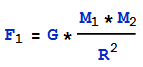This is telling us that the force gravity exerts is equal to the universal gravitational constant times the mass of of the first object, times the mass of the second object, divided by the radius between them squared. For our purposes we will set G=1.4879*10^(-34) in units of AU^3/(kg*Day^2). The reasons for these units is because we want it to work easily with the initial position and velocity data we will acquire from the JPL ephemeris database.

Force can also be expressed as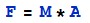. Combining these two equations we find the following: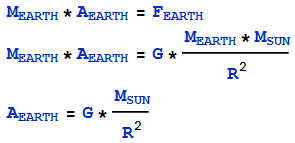Similarly for the Sun we can say: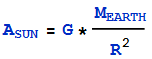So we have found an expression for the acceleration of the sun and the acceleration of Earth. For this simple model we will assume that the amount of movement that the sun experiences from earth is so small it basically stays still. This means we will neglect the acceleration of the sun from our calculations which will make life much simpler. As always, you can add this in later.
Note: Mass sun is a constant, G is a constant, R is NOT a constant

Position and Velocity Data from JPL
JPL keeps a database of the predicted position and velocity of every cataloged object in our solar system. This includes asteroids! We need to select a date, and search the database to tell us our initial conditions which will later be put into Matlab. Go to the JPL HORIZONS web-interface. We only need to find the inial position of the earth for the date specified. This is because we are assuming the sun is at [0,0,0] and has no acceleration or velocity. Make sure the ephemeris type is Vectors. The target body should be Earth (Geocenter) as opposed to the Earth-Moon barycenter which is the center of the Earth-Moon system. We will use a start date of February 12, 2012. A step size of 10 days will give us the position on the 12th and the 22nd.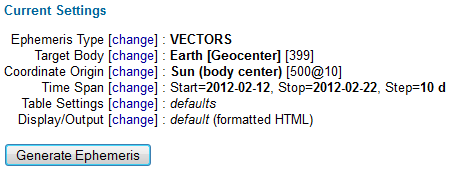JPL HORIZONS Web-Interface

Then we click generate and we get the following data: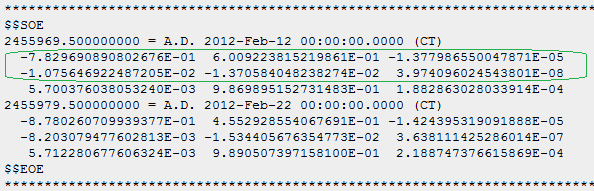The data we will use is highlighted in green. Make sure to read the table at the top of the Ephemeris to make sure you understand what the units are. For our example, in the green box, the top row is the [X,Y,Z] position of Earth in AU. The second row below that is the [Vx,Vy,Vz] velocities of Earth in AU/Day.

Build it in Matlab
Lets review the information we've collected:
• Sun - position, velocity, acceleration all assumed to be zero
• Earth - position and velocity from JPL, have a function for acceleration in terms of radius
Now that we have all our starting information we can build the Matlab simulator. Open up Matlab and create a new script file saved as "SimpleGravity.m". In this we will build our program. We will need to also build a separate function in another script file. Create a new script file and save it as "Propagator.m". SimpleGravity will call Propagator when we run it.

Components of SimpleGravity.m
•  Input the time over which we'd like to see the simulation run as well as the size of the time step we would like to use. A smaller time step means more processing is required.
• Create some global variables
• Input initial conditions for the Sun from our assumptions [0,0,0] position, [0,0,0] velocity
• Input initial conditions for the Earth from JPL
• Call the process ODE45 on the function Propagator.m function
• Plot the results

Components of Propagator.m

This part is where you'll need to look up some Matlab functions and familiarize yourself with Matlab programming. I could walk you through every function call but that wouldn't be exciting. I have included a pdf document detailing the final result of each of the above steps. However, I suggest that you try to write it up yourself first. It's ok if you don't know how the ODE45 command works, just try and get the basic commands down before using the cheat sheet. ODE45 takes your current position, velocity and acceleration and calculates what your next position and velocity will be at some timestep later specified by you. (It uses a Runge-Kutta method for you math geeks). Matlab is wonderfully useful for all sorts of calculations. If you're an engineering student, I really suggest you take the time to really learn and understand this program.

Matlab Code
SimpleGravity.m
Propagator.m

Make sure that you put them in the same directory and execute SimpleGravity.m. Change the result by making the step size smaller or increasing the number of days the final time.

If you want to check how well your simulator works, set finaltime to 10 days. Look at the last line of finalposition, the first three numbers represent the last x,y,z position of Earth. Compare this against the position given by JPL from our initial data show in the picture above.

Feedback
Send Feedback Please! Hope you have been inspired.

## Sunday, February 12, 2012

### Understanding Environmental Impact as a Child

Once upon a time there was a Dragon
And there were no people and there were no trees
The Dragon at all the trees and cows
Then he died in his cave.
.
.
.
The End

This is a story I dictated and illustrated when I was five or six years old and still in preschool. Proof that even children are smart enough to know that when you consume too much it can only lead to disaster.